# Structural Model and Diagram of Multilayer Electro Magneto Elastic Actuator for Nanotechnology

Afonin SM*

National Research University of Electronic Technology, MIET, Moscow, Russia

Received Date: November 10, 2019;  Published Date: November 18, 2019

#### Abstract

We obtained the structural model and the structural diagram of the multilayer electro magneto elastic actuator for nanotechnology in contrast to electrical equivalent circuits of the piezo transmitter and piezo receiver, the vibration piezo motor. We determined the structural model, the structural diagram and the matrix transfer function of the multilayer electro magnetoelastic actuator for nanotechnology.

Keywords: Structural model; Structural diagram; Multilayer electro magneto elastic actuator; Multilayer piezo actuator; Matrix transfer function

#### Introduction

The multilayer electro magneto elastic actuator nano and micro displacement with the piezoelectric, piezomagnetic, electrostriction, magnetostriction effects is used in the control system for nanotechnology in scanning tunneling microscopy, atomic force microscopy and adaptive optics in the range of movement from few nanometers to tens of micrometers [1−32]. We obtained the structural model and the structural diagram of the multilayer electro magneto elastic actuator in contrast to electrical equivalent circuits of the piezo transducer, the vibration piezo motor [1–11]. We used the equation of the electro magneto elasticity, the equivalent mechanical quadrupole and the boundary conditions of the multilayer actuator for the structural model and the structural diagram of the multilayer actuator [8−28]. We received the matrix transfer function of the multilayer electro magneto elastic actuator from its structural model.

#### Introduction

We determined the structural model and the structural diagram of the multilayer electro magneto elastic actuator for nanotechnology and nanomedicine in difference from Cady and Mason electrical equivalent circuits of the piezo transducer. We used the method of the mathematical physics with Laplace transform for the determination the structural model and the structural diagram of the multilayer electro magneto elastic actuator for nanotechnology and nanomedicine [8,14,32].

The equation [8,9,11,25,26] of the electro magneto elasticity has the following form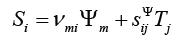Where Si is the relative displacement, ν mi is the coefficient of electro magneto elasticity: dmi piezo module or magneto strictive coefficient, Ψm is control parameter in the form of electric Em , magnetic Hm field strengths or electric Dm induction, sijΨ is the elastic compliance with Ψ = const , Tj is the mechanical stress, i, j, m are the indexes.

We received for the multilayer electro magneto elastic actuator the causes force in the form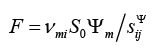where S0 is the cross sectional area of the actuator.

We have matrix the equivalent mechanical quadrupole of the multilayer electro magneto elastic actuator  in the following form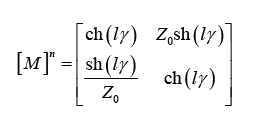where l is length of the multilayer actuator for the longitudinal piezo effect l = nδ , for the transverse piezo effect , for the shift piezo effect l = nb , and δ,h,b are the thickness, the height, the width for the piezo layer.

We obtained the system of the equations for the structural model and the structural diagram of the multilayer electro magneto elastic actuator on Figure 1. We have the structural model of the multilayer electro magneto elastic actuator in result analysis the equation of the force that causes deformation, the equivalent mechanical quadrupole and the boundary conditions equations with the forces on faces of the actuator in the following form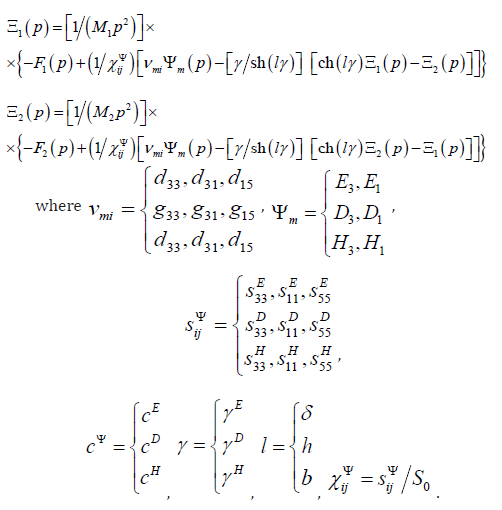From the structural model we have the matrix transfer function of the multilayer electro magneto elastic actuator for nanotechnology in the form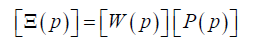where I EQUAare the matrices of the displacements the faces, the transfer functions, and the control parameters.

We have at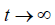the static displacements the faces of the voltage-controlled multilayer piezo actuator for the longitudinal piezo effect and the inertial load for wherem is the mass of the multilayer piezo actuator, M1 ,M2 are the load masses, and the forces on faces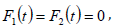in the form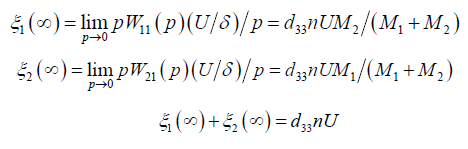where U is the voltage on the actuator.

Therefore, the static displacements the faces of the multilayer piezo actuator at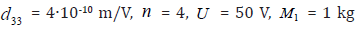and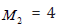we obtained the static displacements of the faces the multilayer piezo actuator#### Conclusion

We obtained the structural model and the structural diagram of the multilayer electro magneto elastic actuator for nanotechnology with the mechanical parameters in the form the displacement and the force in the difference from Cady and Mason electrical equivalent circuits of the piezo transducer.

We determined the structural model, structural diagram and the matrix transfer function of the multilayer electro magneto elastic actuator for nanotechnology.

We received the characteristics of the multilayer electro magneto elastic actuator with using its the matrix transfer function.

None.

#### Conflict of Interest

No conflict of interest.

Article Details
Citation
Keywords
Scroll to Top